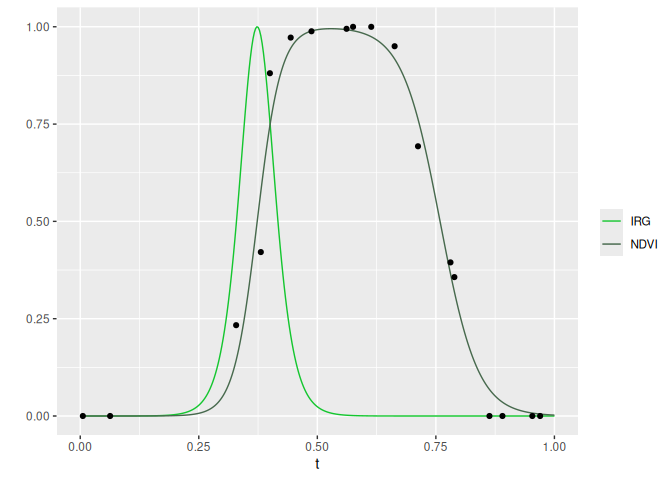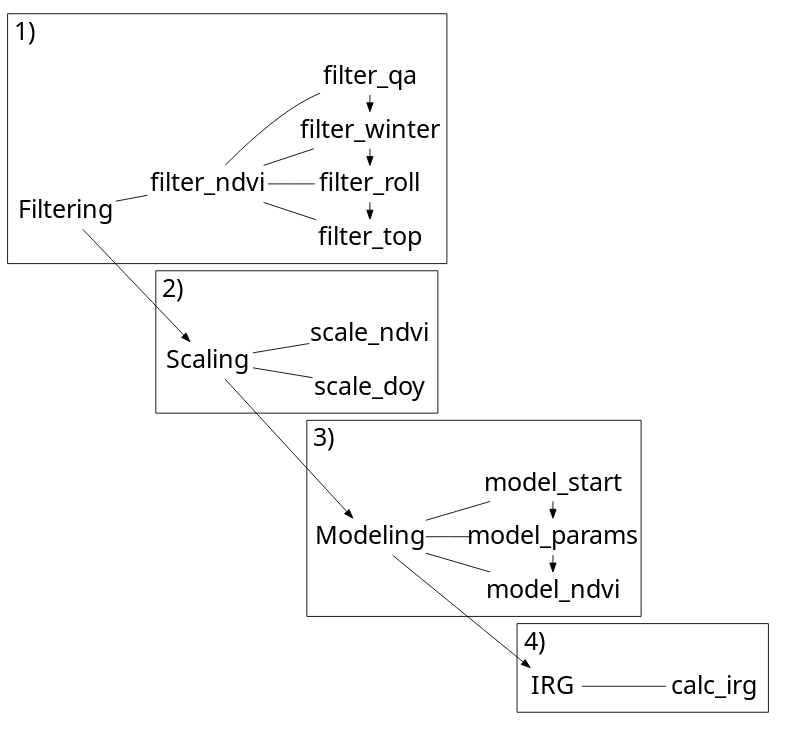irg is an R package for calculating the instantaneous rate of green-up (IRG). It can be used to fit a double logistic curve to a time series of normalized difference vegetation index (NDVI) and calculate IRG, as described in Bischoff et al. (2012) . Remote sensing imagery from MODIS is collected frequently with high temporal resolution (albeit with lower spatial resolution than LANDSAT). IRG helps identify the timing of green-up and can be used to determine if migratory animals are “surfing” a green-wave of high quality forage or if non-migratory animals are selecting available resources at the peak IRG in their environments.

## Approach

The irg package opts for a tabular calculation of IRG as opposed to a raster based approach. Sampling MODIS imagery is left up to the user and a prerequisite for all functions. The main input (DT) for all functions is a data.table of an NDVI time series. The sampling unit (id) is flexible (a decision for the user) though we would anticipate points or polygons, or maybe a pixel. All functions leverage the speed of data.table to efficiently filter, scale, and model NDVI time series and calculate IRG.

More details in the first vignette: Getting started with IRG.

## Installation

Install with CRAN

# Install
install.packages('preparelocs')

or R-universe

# Enable the robitalec universe
options(repos = c(
robitalec = 'https://robitalec.r-universe.dev',
CRAN = 'https://cloud.r-project.org'))

# Install
install.packages('preparelocs')

## Usage

IRG is calculated by filtering an NDVI time series, scaling variables, modeling the time series with a double logistic curve and taking the first derivative of this curve.

Here, we use the meta function irg. Alternatively, use the filtering, scaling, modeling and IRG functions separately (see Getting started with IRG).

library(data.table)
library(ggplot2)
library(irg)

ndvi <- fread(system.file("extdata", "ndvi.csv", package = "irg"))

# Calculate IRG using example data: a raw NDVI time series
IRG <- irg(ndvi)

# Plot IRG and NDVI for 1 year at 1 point (please excuse the manual color scale)
cols <- c('IRG' = '#14c62f', 'NDVI' = '#47694d')
ggplot(IRG[yr == 2007 & id == sample(id, 1)], aes(x = t)) +
geom_line(aes(y = irg, color = 'IRG')) +
geom_line(aes(y = fitted, color = 'NDVI')) +
scale_color_manual(values = cols) +
labs(y = '', color = '')## Functions## Contributing

Contributions welcome! See details in CONTRIBUTING.md.

Please note that the irg package is released with a Contributor Code of Conduct. By contributing to this package, you agree to abide by its terms.

## Thanks

To Mike Laforge (@MamlSpatialEco) and Eric Vander Wal for thoughtful discussion that stimulated development of this package.

 Bischof, R., Loe, L. E., Meisingset, E. L., Zimmermann, B., Van Moorter, B., & Mysterud, A. (2012). A migratory northern ungulate in the pursuit of spring: jumping or surfing the green wave? The American Naturalist, 180(4), 407-424.